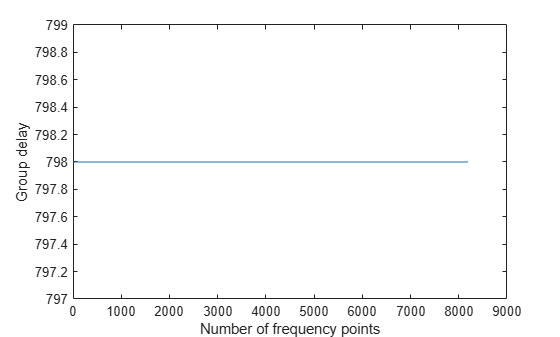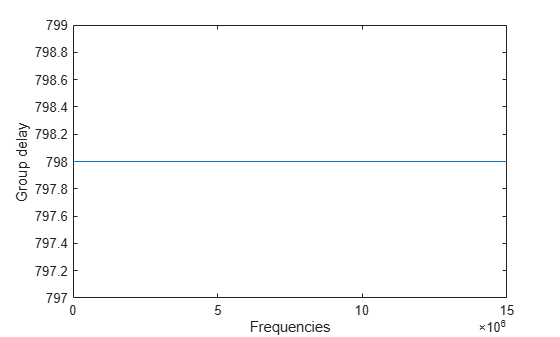Documentation

groupDelay

Group delay of digital down converter or digital up converter filter cascade

Description

D = groupDelay(Conv,N) returns a vector of group delays, D, of a digital down converter or digital up converter, Conv, evaluated at N frequency points. The frequency points are equally spaced around the upper half of the unit circle.

example

[D,F] = groupDelay(Conv,N) returns a vector of frequencies, F, at which the group delay has been computed.

Examples

collapse all

Compute the group delays of the digital down converter using the groupDelay function.

Create a dsp.DigitalDownConverter System object with the default settings.

dwnConv = dsp.DigitalDownConverter
dwnConv =
dsp.DigitalDownConverter with properties:

DecimationFactor: 100
MinimumOrderDesign: true
Bandwidth: 200000
StopbandFrequencySource: 'Auto'
PassbandRipple: 0.1000
StopbandAttenuation: 60
Oscillator: 'Sine wave'
CenterFrequency: 14000000
SampleRate: 30000000

Show all properties

Use the groupDelay function to compute the vector of group delays. By default, the function evaluates the group delays at 8192 frequency points equally spaced around the upper half of the unit circle.

D = groupDelay(dwnConv);

Plot the vector of group delays.

plot(D)
xlabel('Number of frequency points')
ylabel('Group delay')Determine the vector of frequencies over which the group delays are computed and plot them.

[D,F] = groupDelay(dwnConv);
plot(F,D)
xlabel('Frequencies')
ylabel('Group delay')Input Arguments

collapse all

Digital down converter or digital up converter, specified as a dsp.DigitalDownConverter or dsp.DigitalUpConverter System object™.

Number of frequency points over which the group delays are evaluated, specified as a positive scalar. These points are equally spaced around the upper half of the unit circle.

Data Types: double | single

Output Arguments

collapse all

Vector of group delays of the digital down converter or digital up converter, evaluated at N frequency points equally spaced around the upper half of the unit circle.

Data Types: double

Frequencies at which the group delays are evaluated, returned as a column vector.

Data Types: double

Watch now